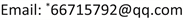Understanding and Thinking about Fractional Arithmetic and Its Related Contents

—Taking the Comparison of Textbooks between PEP and BNUP as an Example

Zhen Tang, Lin Chen*

College of Mathematics and Statistics, Yili Normal University, Yining XinjiangReceived: Jun. 12th, 2021; accepted: Jul. 9th, 2021; published: Jul. 15th, 2021ABSTRACT

Fractional arithmetic is the key and difficult part of “Number and Algebra” in primary school mathematics, and it is also an important expansion of the concept of number. This paper adopts content analysis method and literature research method, taking textbooks of PEP and BNUP as examples, conducts a comparative study on the content related to fractional arithmetic from many aspects, and the results provide some theoretical reference for further textbook reform in the future.

Keywords:Fractional Arithmetic, PEP, BNUP, The Comparison of Textbooks1. 引言

2. 研究方法及对象2.1. 研究方法

2.2. 研究对象

3. 研究结果3.1. 内容结构对比

PEP edition primary school mathematics textbook (PEP

Beijing Normal University version of elementary school mathematics textbook (BNUP

3.2. 引入对比

3.3. 总结

“新疆高校科研计划重点项目”(XJEDU2016I043)；“伊犁师范大学博士科研启动基金项目”(2017YSBS08)。